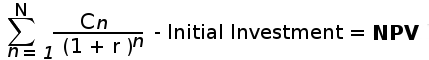# NPV vs IRR : Which is better for capital budgeting?

NPV (Net Present Value) and IRR (Internal Rate Of Return) are the two most important financial metric in capital budgeting, which are used to evaluate the profitability of a project in future.

NPV, in simple terms, is the difference between the present value of cash inflows and the present value of cash outflows. A positive NPV value is likely to yield profit in future for an investment or a project, whereas, a negative NPV value is expected to bring loss.

The formula for calculating NPV is as follows:Where in the above formula :

N = total number of periods

n = positive integer

C = cash flow

r = discount rate

NPV = net present value

IRR is expressed in terms of NPV. IRR is the discount rate (r) that makes the NPV of all cash flows from a particular project equal to zero. If the IRR is more than the company's minimum acceptable rate of return then the project is favorable else it's not.

None of these two financial entities are perfect for evaluating the future outcome. Each one of these two has it's own advantages and disadvantages. Let's compare them and also find out which is better.

## Comparison and difference

1. NPV is expressed in form of cash return value, where as the IRR is expressed in percentage.

2. NPV measure is absolute but IRR measure is relative. For example, an IRR of 20% may or may not be acceptable. The out-coming IRR is first compared with the company's own acceptable rate of return. That's why IRR is a relative term.

3. IRR is not applicable to evaluate a project or investment where cash flow is changing over time. In such cases, NPV is more appropriate as it takes each cash flows into it's consideration.

4. In the long-term project the discount rate is more likely to change, which makes IRR less valuable financial metric than NPV.

5. Decision making is easier in NPV than IRR. In the case of NPV, any positive value (NPV > 0) is considered as acceptable and likely to add value to the company. Where as, in IRR, the rate of return is compared with the companies own acceptable rate of return. Which makes the decision further difficult.

6. The NPV takes into account any additional wealth for calculating profitability but IRR method does not.

7. When the initial investment is very high, the NPV will show large cash inflows. In this case, IRR will show better result as it reflects the percentage return irrespective of the initial cash flow.

8. Financial analyst prefer IRR in short-term individual projects but for the long-term mutually exclusive projects they prefer NPV.

## Conclusion

Overall, NPV is a better financial tool for capital budgeting as it takes into account the variation in cash-flow, discount rate and additional wealth. which sounds more theoretical and realistic.